Courses

# Car Following Models Civil Engineering (CE) Notes | EduRev

## Civil Engineering (CE) : Car Following Models Civil Engineering (CE) Notes | EduRev

The document Car Following Models Civil Engineering (CE) Notes | EduRev is a part of the Civil Engineering (CE) Course Transportation Engineering.
All you need of Civil Engineering (CE) at this link: Civil Engineering (CE)

Car following models

Car following theories describe how one vehicle follows another vehicle in an uninterrupted flow. Various models were formulated to represent how a driver reacts to the changes in the relative positions of the vehicle ahead. Models like Pipes, Forbes, General Motors and Optimal velocity model are worth discussing. 14.2.1 Notation Before going in to the details, various notations used in car-following models are discussed here with the help of figure 14:1. The leader vehicle is denoted as n and the following vehicle as (n+1). Two characteristics at an instant t are of importance; location and speed. Location and speed of the lead vehicle at time instant t are represented by x t n and v t n respectively. Similarly, the location and speed of the follower are denoted by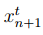and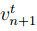respectively. The following vehicle is assumed to accelerate at time t + ∆T and not at t, where ∆T is the interval of time required for a driver to react to a changing situation. The gap between the leader and the follower vehicle is therefore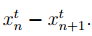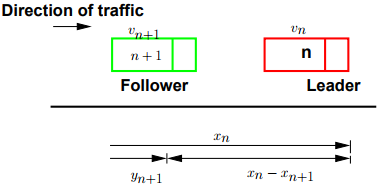Figure 14:1: Notation for car following model

Pipe’s model

The basic assumption of this model is “A good rule for following another vehicle at a safe distance is to allow yourself at least the length of a car between your vehicle and the vehicle ahead for every ten miles per hour of speed at which you are traveling” According to Pipe’s car-following model, the minimum safe distance headway increases linearly with speed. A disadvantage of this model is that at low speeds, the minimum headways proposed by the theory are considerably less than the corresponding field measurements.

Forbes’ model

In this model, the reaction time needed for the following vehicle to perceive the need to decelerate and apply the brakes is considered. That is, the time gap between the rear of the leader and the front of the follower should always be equal to or greater than the reaction time. Therefore, the minimum time headway is equal to the reaction time (minimum time gap) and the time required for the lead vehicle to traverse a distance equivalent to its length. A disadvantage of this model is that, similar to Pipe’s model, there is a wide difference in the minimum distance headway at low and high speeds.

General Motors’

model The General Motors’ model is the most popular of the car-following theories because of the following reasons:

1. Agreement with field data; the simulation models developed based on General motors’ car following models shows good correlation to the field data.

2. Mathematical relation to macroscopic model; Greenberg’s logarithmic model for speeddensity relationship can be derived from General motors car following model.

In car following models, the motion of individual vehicle is governed by an equation, which is analogous to the Newton’s Laws of motion. In Newtonian mechanics, acceleration can be regarded as the response of the particle to stimulus it receives in the form of force which includes both the external force as well as those arising from the interaction with all other particles in the system. This model is the widely used and will be discussed in detail later.

Optimal velocity model

The concept of this model is that each driver tries to achieve an optimal velocity based on the distance to the preceding vehicle and the speed difference between the vehicles. This was an alternative possibility explored recently in car-following models. The formulation is based on the assumption that the desired speed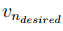depends on the distance headway of the nth vehicle. i.e.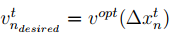where  vopt  is the optimal velocity function which is a function of the instantaneous distance headway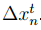Therefore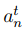is given by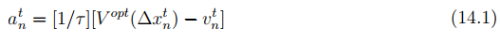where 1/T is called as sensitivity coefficient. In short, the driving strategy of n th vehicle is that, it tries to maintain a safe speed which in turn depends on the relative position, rather than relative speed.

General motor’s car following model

Basic Philosophy

The basic philosophy of car following model is from Newtonian mechanics, where the acceleration may be regarded as the response of a matter to the stimulus it receives in the form of the force it receives from the interaction with other particles in the system. Hence, the basic philosophy of car-following theories can be summarized by the following equation

[Response]n α [Stimulus]n                                                                                         (14.2)

for the nth vehicle (n=1, 2, ...). Each driver can respond to the surrounding traffic conditions only by accelerating or decelerating the vehicle. As mentioned earlier, different theories on carfollowing have arisen because of the difference in views regarding the nature of the stimulus. The stimulus may be composed of the speed of the vehicle, relative speeds, distance headway etc, and hence, it is not a single variable, but a function and can be represented as,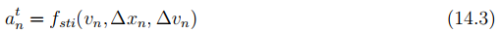where fsti is the stimulus function that depends on the speed of the current vehicle, relative position and speed with the front vehicle.

The car following model proposed by General motors is based on follow-the leader concept. This is based on two assumptions; (a) higher the speed of the vehicle, higher will be the spacing between the vehicles and (b) to avoid collision, driver must maintain a safe distance with the vehicle ahead.

Let ∆+1 is the gap available for (n + 1)th vehicle, and let ∆xsafe is the safe distance,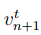and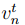are the velocities, the gap required is given by,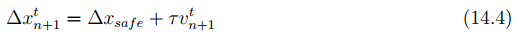where τ is a sensitivity coefficient. The above equation can be written as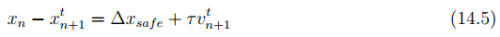Differentiating the above equation with respect to time, we get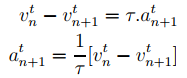General Motors has proposed various forms of sensitivity coefficient term resulting in five generations of models. The most general model has the form,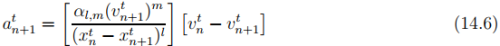where l is a distance headway exponent and can take values from +4 to -1, m is a speed exponent and can take values from -2 to +2, and α is a sensitivity coefficient. These parameters are to be calibrated using field data. This equation is the core of traffic simulation models.

In computer, implementation of the simulation models, three things need to be remembered:

1. A driver will react to the change in speed of the front vehicle after a time gap called the reaction time during which the follower perceives the change in speed and react to it.

2. The vehicle position, speed and acceleration will be updated at certain time intervals depending on the accuracy required. Lower the time interval, higher the accuracy.

3. Vehicle position and speed is governed by Newton’s laws of motion, and the acceleration is governed by the car following model.

Therefore, the governing equations of a traffic flow can be developed as below. Let ∆T is the reaction time, and ∆t is the updation time, the governing equations can be written as,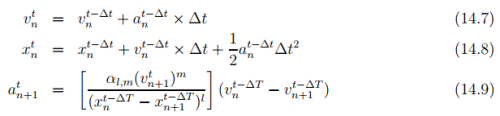The equation 14.7 is a simulation version of the Newton’s simple law of motion v = u + at and equation 14.8 is the simulation version of the Newton’s another equation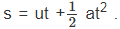. The acceleration of the follower vehicle depends upon the relative velocity of the leader and the follower vehicle, sensitivity coefficient and the gap between the vehicles.

Numerical Example Let a leader vehicle is moving with zero acceleration for two seconds from time zero. Then he accelerates by 1 m/s2 for 2 seconds, then decelerates by 1m/s2 for 2 seconds. The initial speed is 16 m/s and initial location is 28 m from datum. A vehicle is following this vehicle with initial speed 16 m/s, and position zero. Simulate the behavior of the following vehicle using General Motors’ Car following model (acceleration, speed and position) for 7.5 seconds. Assume the parameters l=1, m=0 , sensitivity coefficient (αl,m) = 13, reaction time as 1 second and scan interval as 0.5 seconds.

Solution The first column shows the time in seconds. Column 2, 3, and 4 shows the acceleration, velocity and distance of the leader vehicle. Column 5,6, and 7 shows the acceleration, velocity and distance of the follower vehicle. Column 8 gives the difference in velocities between the leader and follower vehicle denoted as dv. Column 9 gives the difference in displacement between the leader and follower vehicle denoted as dx. Note that the values are assumed to be the state at the beginning of that time interval. At time t=0, leader vehicle has a velocity of 16 m/s and located at a distance of 28 m from a datum. The follower vehicle is also having the same velocity of 16 m/s and located at the datum. Since the velocity is same for both, dv = 0. At time t = 0, the leader vehicle is having acceleration zero, and hence has the same speed. The location of the leader vehicle can be found out from equation as, x = 28+16×0.5 = 36 m. Similarly, the follower vehicle is not accelerating and is maintaining the same speed. The location of the follower vehicle is, x = 0+16×0.5 = 8 m. Therefore, dx = 36-8 =28m. These steps are repeated till t = 1.5 seconds. At time t = 2 seconds, leader vehicle accelerates at the rate of 1 m/s2 and continues to accelerate for 2 seconds. After that it decelerates for a period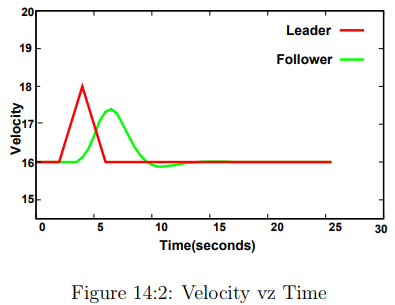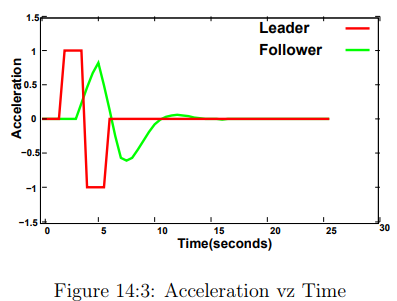of two seconds. At t= 2.5 seconds, velocity of leader vehicle changes to 16.5 m/s. Thus dv becomes 0.5 m/s at 2.5 seconds. dx also changes since the position of leader changes. Since the reaction time is 1 second, the follower will react to the leader’s change in acceleration at 2.0 seconds only after 3 seconds. Therefore, at t=3.5 seconds, the follower responds to the leaders change in acceleration given by equation i.e.,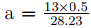= 0.23 m/s2 . That is the current acceleration of the follower vehicle depends on dv and reaction time ∆ of 1 second. The follower will change the speed at the next time interval. i.e., at time t = 4 seconds. The speed of the follower vehicle at t = 4 seconds is given by equation as v= 16+0.231×0.5 = 16.12 The location of the follower vehicle at t = 4 seconds is given by equation as x = 56+16×0.5+1 2×0.231×0.5 2 = 64.03 These steps are followed for all the cells of the table. The earliest car-following models considered the difference in speeds between the leader and the follower as the stimulus. It was assumed that every driver tends to move with the same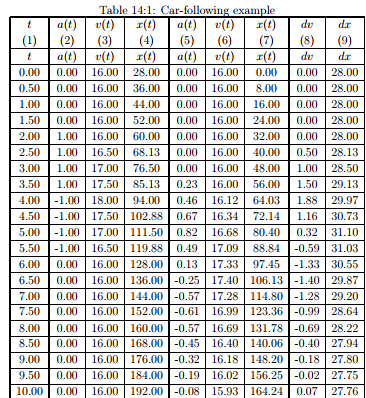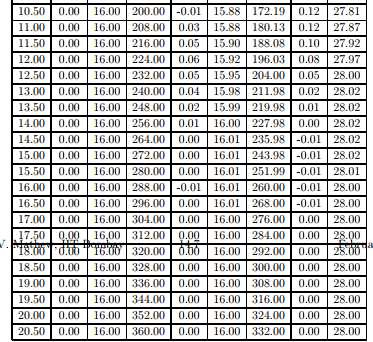speed as that of the corresponding leading vehicle so that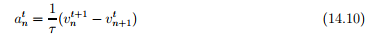where τ is a parameter that sets the time scale of the model and 1/r can be considered as a measure of the sensitivity of the driver. According to such models, the driving strategy is to follow the leader and, therefore, such car-following models are collectively referred to as the follow the leader model. Efforts to develop this stimulus function led to five generations of car-following models, and the most general model is expressed mathematically as follows.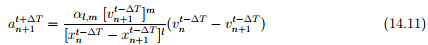where l is a distance headway exponent and can take values from +4 to -1, m is a speed exponent and can take values from -2 to +2, and α is a sensitivity coefficient. These parameters are to be calibrated using field data.

Offer running on EduRev: Apply code STAYHOME200 to get INR 200 off on our premium plan EduRev Infinity!

## Transportation Engineering

23 videos|66 docs|39 tests

,

,

,

,

,

,

,

,

,

,

,

,

,

,

,

,

,

,

,

,

,

;